# Contour Plots of Matrix Data

This page shows how to use R to draw a table or matrix of numerical values as a contour plot with an overlayed grid, like the image below, and using level-plots as an alternative.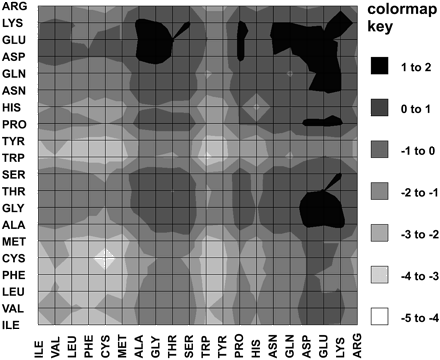This plot is Figure 2 from Lu et al 2003, and shows their residue based "Protein-Protein Interaction Potential" between each of the twenty amino acids.

Contour plots are usually used for continuous variables (e.g. latitude and longitude) rather than categorical data (like amino acid type) as in this case.

## The Data

The data shown in this figure is available as a simple table, MULTIPOT_lu.txt. If you download this file, you can load it into R like so:

What does this data look like? Well its a 20 by 20 numeric matrix:

```> dim(potentials)
 20 20
> rownames(potentials)
 "GLY" "ALA" "SER" "CYS" "VAL" "THR" "ILE" "PRO" "MET" "ASP"
 "ASN" "LEU" "LYS" "GLU" "GLN" "ARG" "HIS" "PHE" "TYR" "TRP"
> colnames(potentials)
 "GLY" "ALA" "SER" "CYS" "VAL" "THR" "ILE" "PRO" "MET" "ASP"
 "ASN" "LEU" "LYS" "GLU" "GLN" "ARG" "HIS" "PHE" "TYR" "TRP"
> min(potentials)
 -4.4
> max(potentials)
 1.9
> potentials["CYS","CYS"]
 -4.4```

## Simple Contour Plot

Now lets use the filled.contour function from the gplots package to display this:

library(gplots)
filled.contour(potentials, main="Protein-Protein Interaction Potential")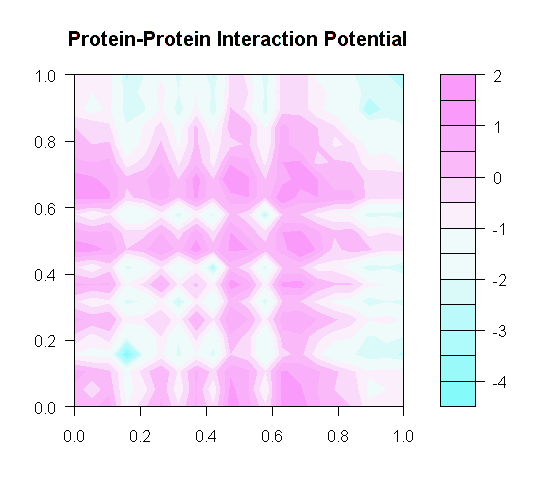Well, I don't care for the default pink and blue colour scheme, but more importantly, notice the axes labels run from 0.0 to 1.0, rather than using the row and column names (i.e. three letter amino acid codes). Trying filled.contour(potentials, axes=FALSE, frame.plot=TRUE) will get rid of the axes labels - but you also loose the key axes.

## Labeling the Axes

There may be a more concise method, but I wrote my own axes drawing function (which calls axis() twice), and supplied this to the filled.contour() function using the plot.axes argument:

matrix.axes <- function(data) {
# Do the rows, las=2 for text perpendicular to the axis
x <- (1:dim(data) - 1) / (dim(data) - 1);
axis(side=1, at=x, labels=rownames(data), las=2);
# Do the columns
x <- (1:dim(data) - 1) / (dim(data) - 1);
axis(side=2, at=x, labels=colnames(data), las=2);
}
filled.contour(potentials, plot.axes=matrix.axes(potentials), main="Protein-Protein Interaction Potential")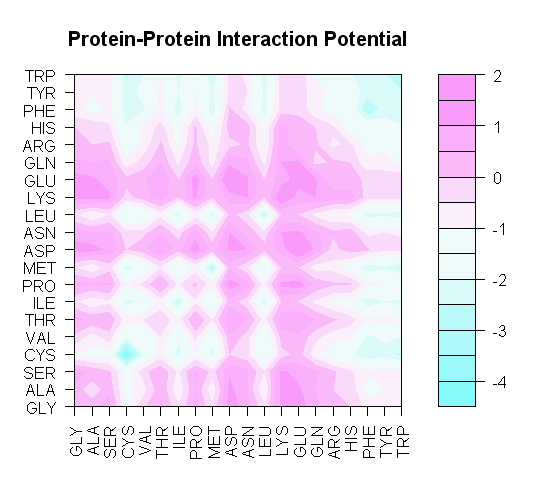Much nicer. This brings out another issue - to match the published figure, we need to reorder our matrix...

## Permuting the Matrix

I re-ordered the matrix like so:

new.order <- c("ILE", "VAL", "LEU", "PHE", "CYS", "MET", "ALA", "GLY", "THR", "SER", "TRP", "TYR", "PRO", "HIS", "ASN", "GLN", "ASP", "GLU", "LYS", "ARG")
pots2 <- potentials[new.order, new.order]
filled.contour(pots2, plot.axes=matrix.axes(pots2), main="Protein-Protein Interaction Potential")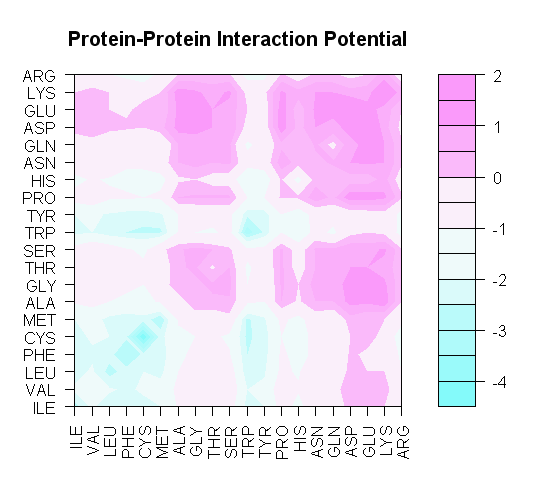## Switching the Colours

We can use the gplots package's function colorpanel() to generate a suitable grey-scale colour scheme to mimic the published figure. I have used seven levels, and choosen to range from white to grey10 (a dark grey):

> library(gplots)
> colorpanel(7, "white", "grey10")
 "#FFFFFF" "#D9D9D9" "#B3B3B3" "#8D8D8D" "#666666" "#404040" "#1A1A1A"

As you can see, that gives seven colours (as hexidemical RGB strings). We can pass that to the filled.contour function like this:

filled.contour(pots2, plot.axes=matrix.axes(pots2), col=colorpanel(7, "white", "grey10"), nlevels=7, main="Protein-Protein Interaction Potential")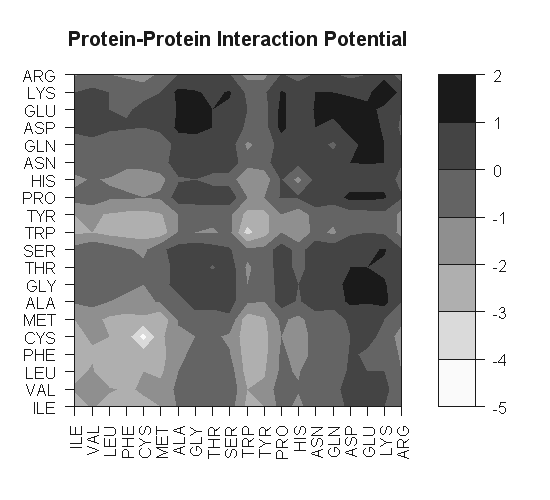Apart from the key layout, the only remaining big difference to the published figure is we don't have the grid lines in our plot... let's have a go at fixing this with the grid() command:

matrix.axes <- function(data) {
# Do the rows, las=2 for text perpendicular to the axis
x <- (1:dim(data) - 1) / (dim(data) - 1);
axis(side=1, at=x, labels=rownames(data), las=2);
# Do the columns
x <- (1:dim(data) - 1) / (dim(data) - 1);
axis(side=2, at=x, labels=colnames(data), las=2);
# Add a solid black grid
grid(nx=(dim(data)-1), ny=(dim(data)-1), col="black", lty="solid");
}
filled.contour(pots2, plot.axes=matrix.axes(pots2), col=colorpanel(7, "white", "grey10"), nlevels=7, main="Protein-Protein Interaction Potential")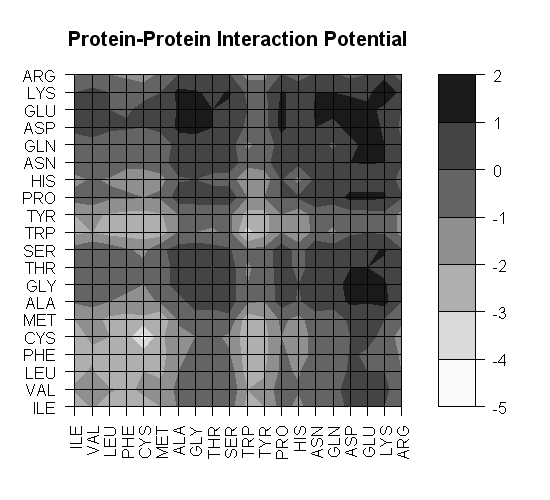That's now looking pretty close, but if you look carefully the published figure seems to determine its contour lines slightly differently:## Discussion

Looking at their Figure 2 does beg the question, why did Lu et al. choose to display the amino acids in this order? Quoting from their paper (see references):

The 20×20 residue-based potential constructed from DIMER- 1 is plotted in Fig. 2. Hydrophobic residues are attractive to each other and hydrophilic residues are repulsive. For example, Leu has an attractive potential with all residues except Lys, Glu, and Asp. Besides the Cys-Cys pair that may form a disulfide bond, the most attractive pairs are between hydrophobic residues pairs such as Leu, Ilu, and Phe. The most repulsive pairs are Asp-Asp, Lys-Lys, Glu- Glu, Glu-Gly, and Asp-Gly. This pattern is similar to what has been observed in the statistical potential obtained from monomers.

Given this, a sorting based on hydrophobicity would seem sensible. According to Shaun Black, the trend for physiological L-alpha-Amino Acids is: Phe > Leu = Ile > Tyr = Trp > Val > Met > Pro > Cys > Ala > Gly > Thr > Ser > Lys > Gln > Asn > His > Glu > Asp > Arg. Thus:

hydro.order <- c("PHE", "LEU", "ILE", "TYR", "TRP", "VAL", "MET", "PRO", "CYS", "ALA", "GLY", "THR", "SER", "LYS", "GLN", "ASN", "HIS", "GLU", "ASP", "ARG")
pots3 <- potentials[hydro.order, hydro.order]
filled.contour(pots3, plot.axes=matrix.axes(pots3), col=colorpanel(7, "white", "grey10"), nlevels=7, main="Protein-Protein Interaction Potential")This does show that hydrophobicity seems to account for much of the potential, with Cys and His perhaps as special cases. Tweaking this order by hand its possible to get a much more eye-pleasing graph.

For the sake of argument, what ordering does the data itself suggest? We could try this using a heatmap, see this page for details:

heatmap.2(pots2, scale="none", breaks=-5:2, col=colorpanel(7, "white", "grey10"), key=TRUE, symkey=FALSE, density.info="none", trace="none")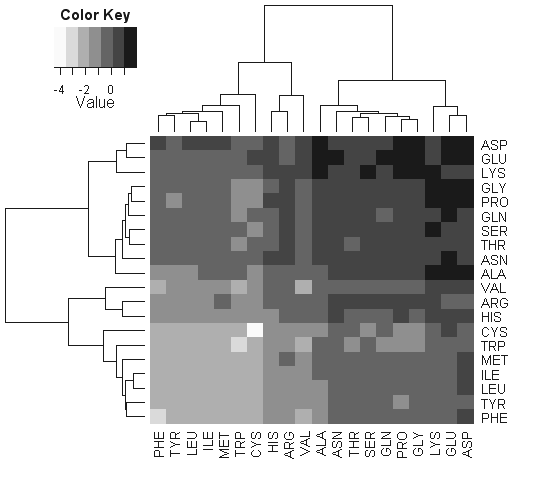## Level Plots

The above heatmap image brings up another point - are contour plots really suitable for a categorical matrix? What about a "level plot" instead? Internally the heatmap functions use the image() function. As before, getting the axis labelled with the row/column names takes a little work...

library(gplots) # for colorpanel()
nr <- dim(pots2)
nc <- dim(pots2)
#Request a square plot area:
par(pty="s")
#Plot an image using the row/column numbers as the x/y variables:
image(x=1:nr, y=1:nc, z=pots2, axes=FALSE, frame.plot=TRUE, breaks=-5:2, col=colorpanel(7, "white", "grey10"), main="Protein-Protein Interaction Potential", xlab=NA, ylab=NA)
#Add axis with the amino acids names, use las=2 for perpendicular text:
axis(1, 1:nr, labels=rownames(pots2), las=2)
axis(2, 1:nc, labels=colnames(pots2), las=2)
#Get a nice border round the image:
box()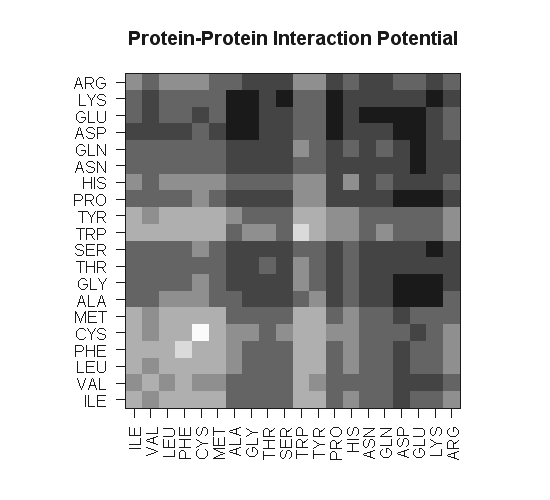grid(nx=nr, ny=nc, col="black", lty="solid")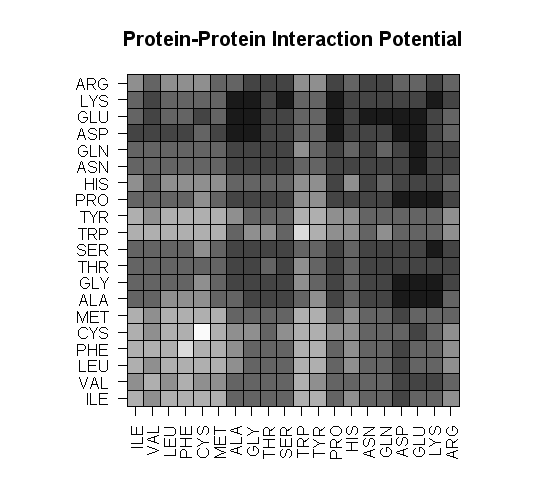As an alternative, we can use the levelplot() function in the lattice package. I have tweaked the tick marks (to turn them off) and rotated the x-axis labels:

library(gplots) # for colorpanel()
library(lattice) # for levelplot()
levelplot(pots2, scales=list(tck=0, x=list(rot=90)), col.regions=colorpanel(7, "white", "grey10"), at=c(-5,-4,-3,-2,-1,0,1,2), main="Protein-Protein Interaction Potential", xlab=NULL, ylab=NULL)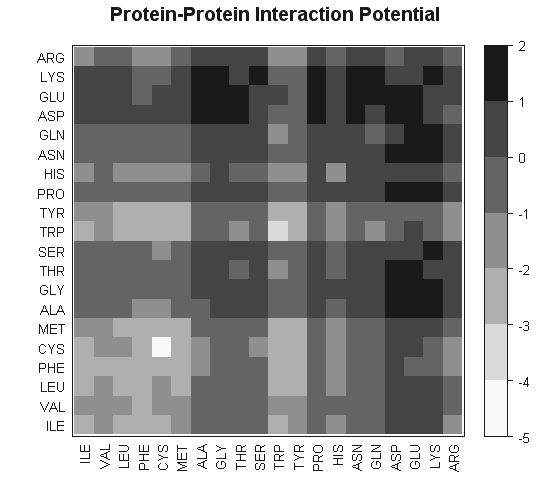One subtle difference between these figures (i.e. image() versus levelplot()) is the treatment of values exactly on the thresholds (e.g. PHE-PHE or VAL-VAL).

P.S. If you use levelplot() inside your own functions, you have to use print(levelplot(...)) to actually produce the graph. See this R-FAQ entry.

## References

Lu et al. (2003) Development of Unified Statistical Potentials Describing Protein-Protein Interactions, Biophysical Journal 84(3), p1895-1901 (PMID: 12609891, journal link, supplementary data)